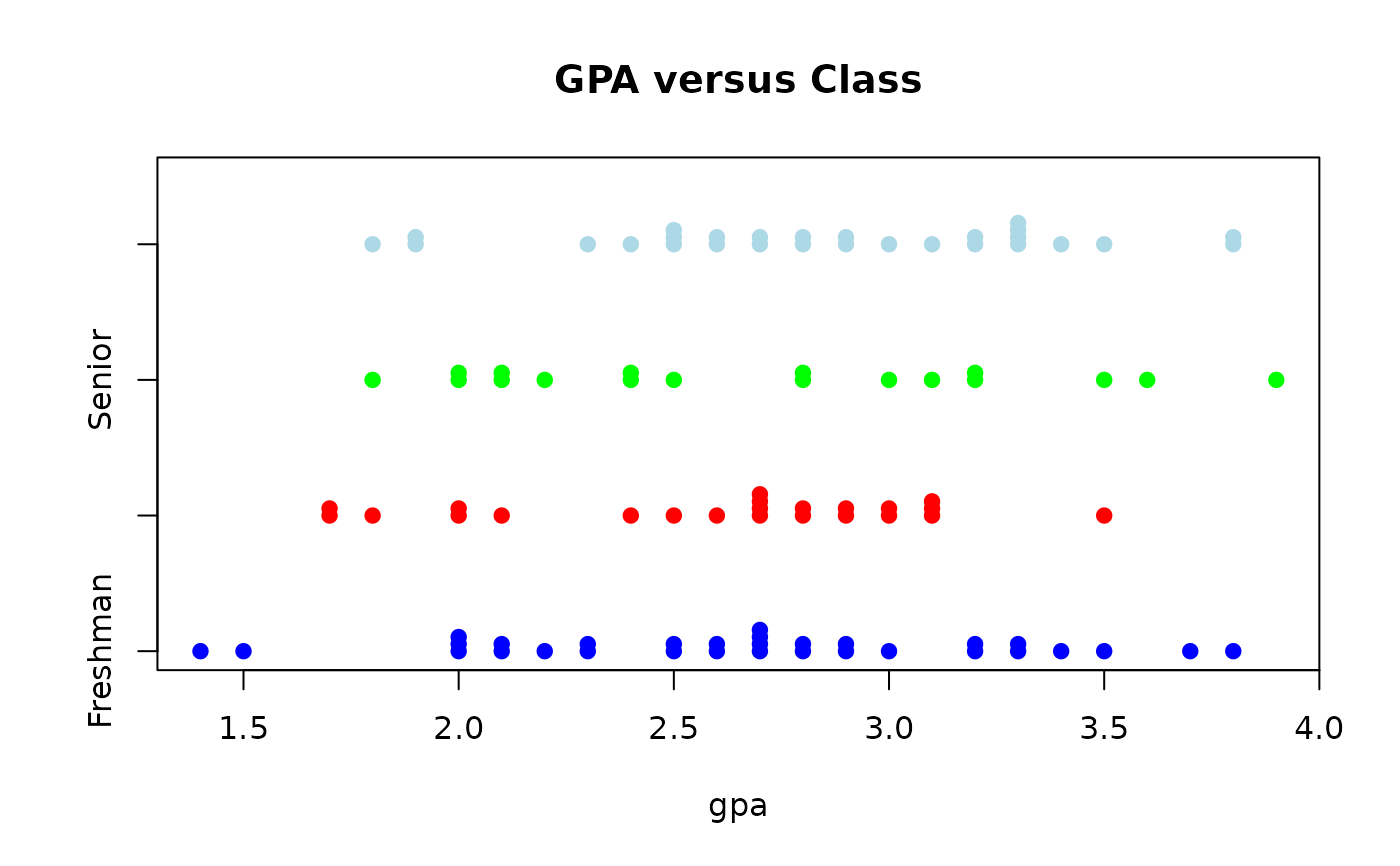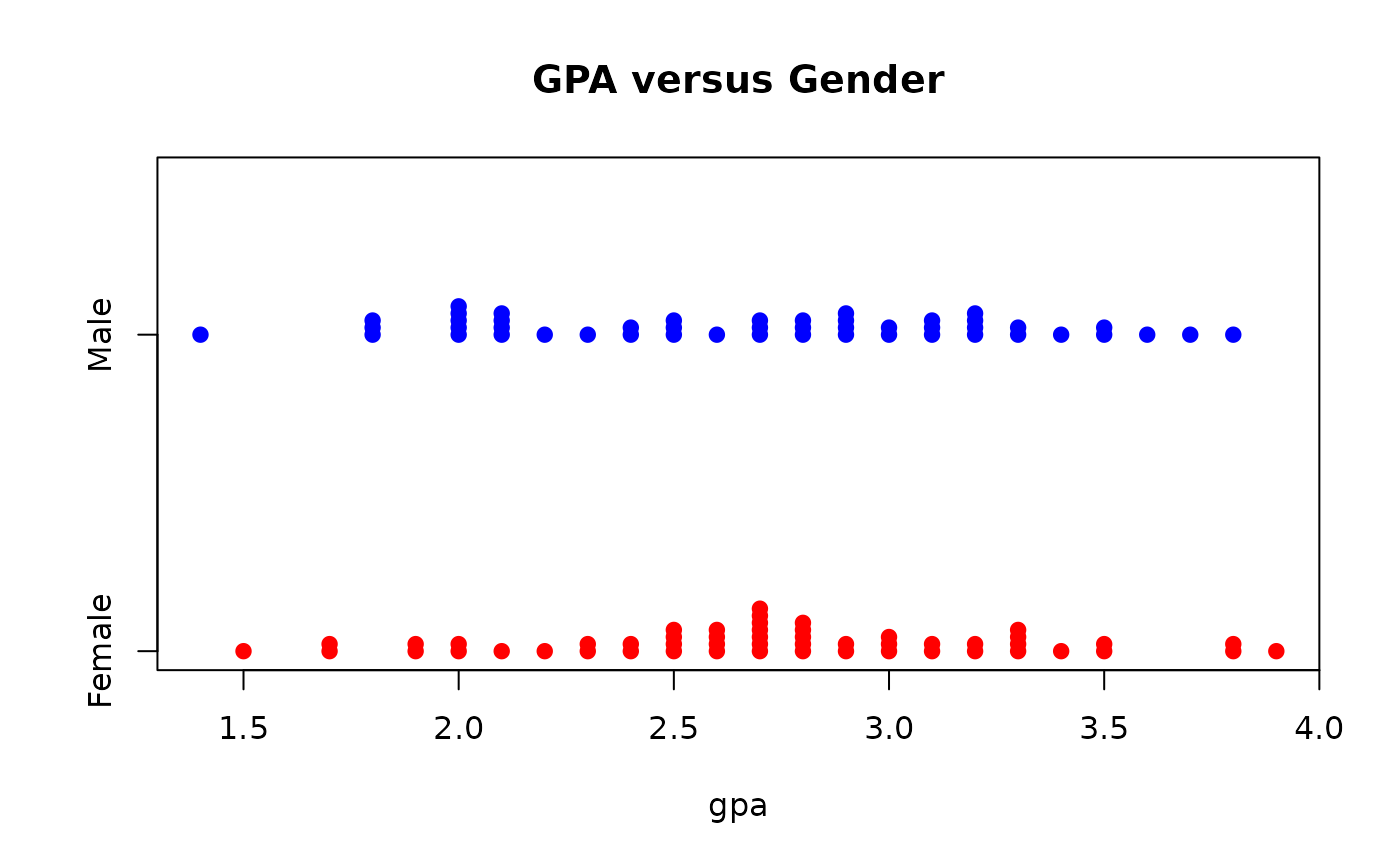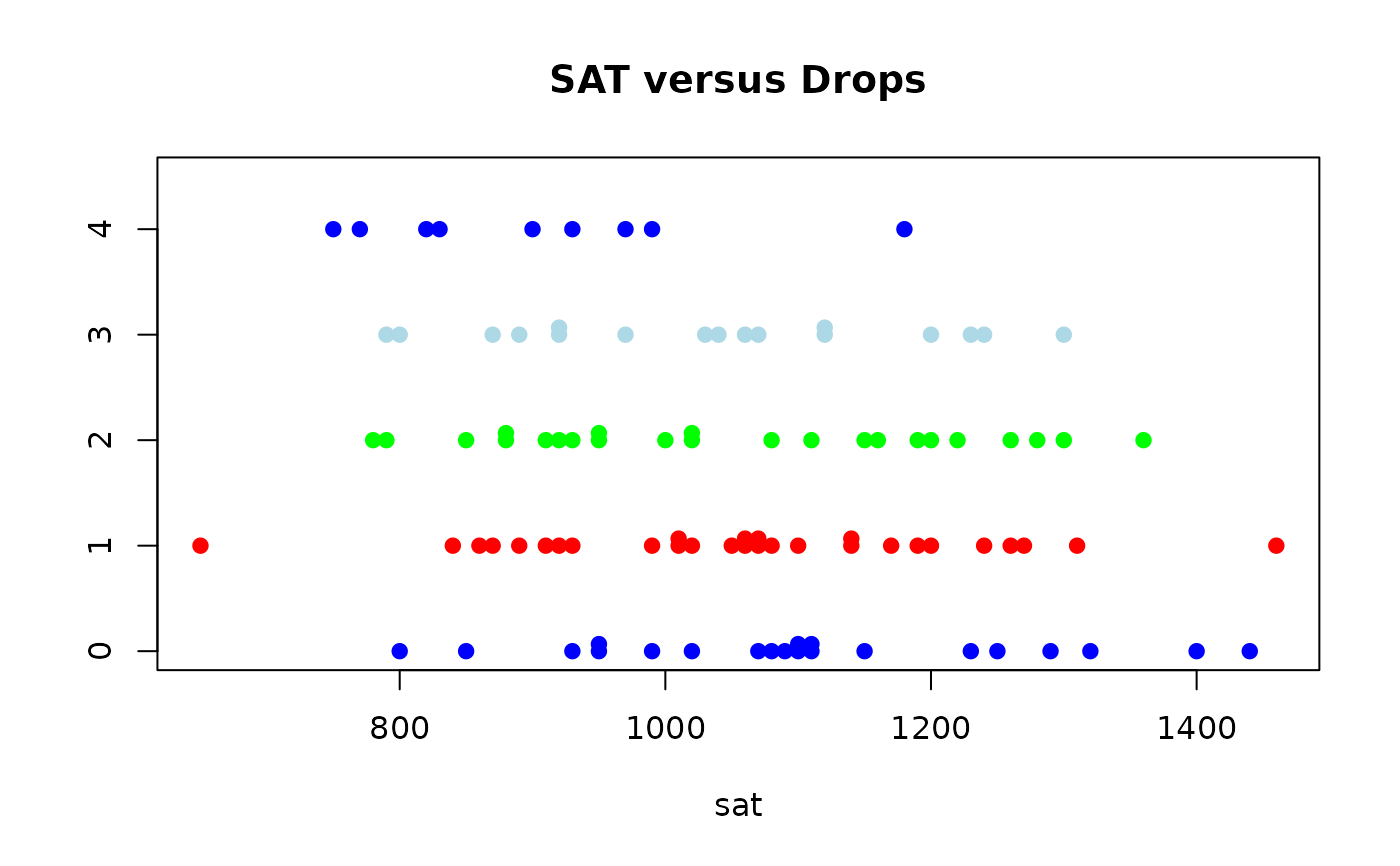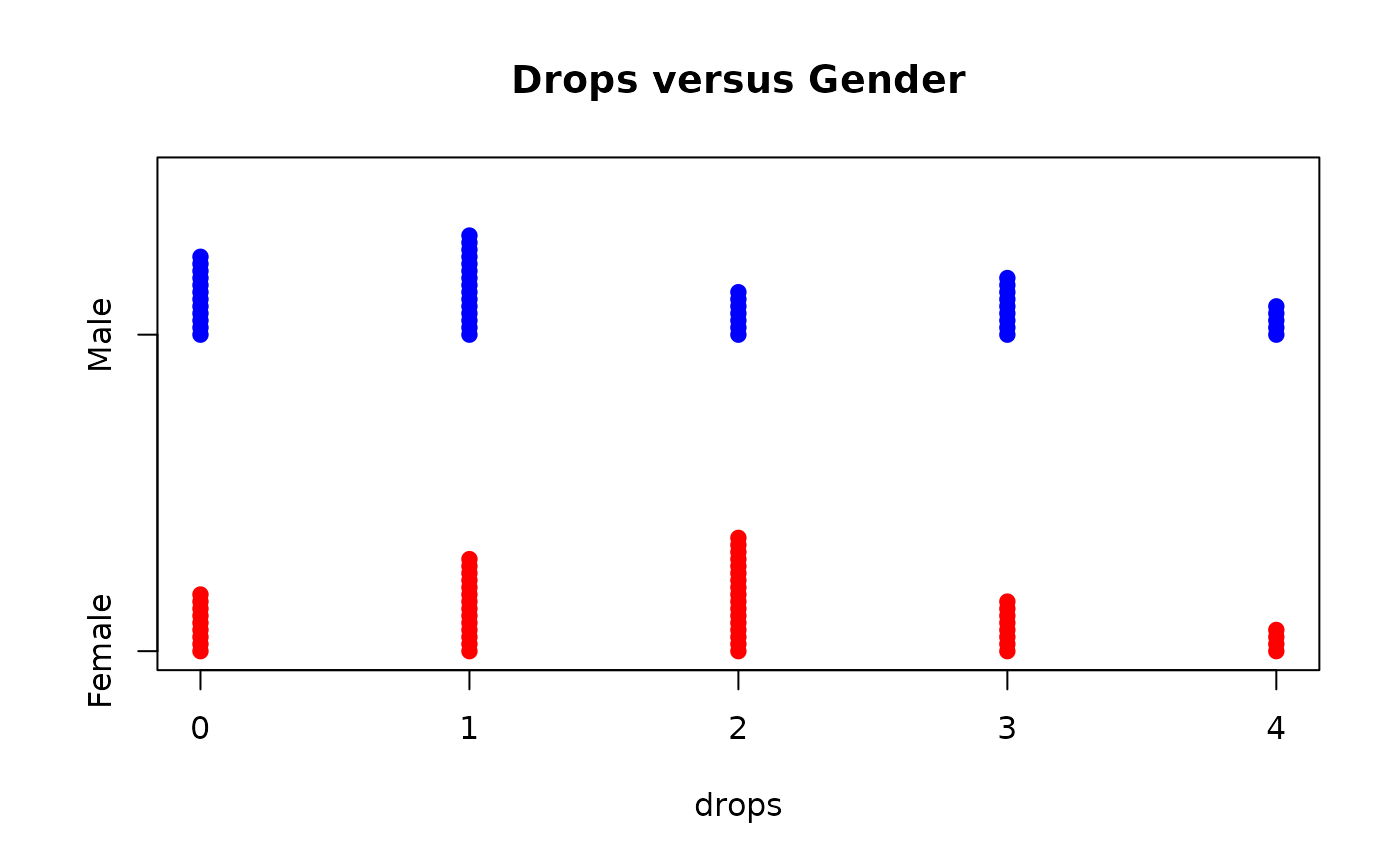Data for Exercise 1.15

Undergrad

## Format

A data frame/tibble with 100 observations on six variables

gender

character variable with values Female and Male

major

college major

class

college year group classification

gpa

sat

Scholastic Assessment Test score

drops

number of courses dropped

## Examples


stripchart(gpa ~ class, data = Undergrad, method = "stack",
col = c("blue","red","green","lightblue"),
pch = 19, main = "GPA versus Class")stripchart(gpa ~ gender, data = Undergrad, method = "stack",
col = c("red", "blue"), pch = 19,
main = "GPA versus Gender")stripchart(sat ~ drops, data = Undergrad, method = "stack",
col = c("blue", "red", "green", "lightblue"),
pch = 19, main = "SAT versus Drops")stripchart(drops ~ gender, data = Undergrad, method = "stack",
col = c("red", "blue"), pch = 19, main = "Drops versus Gender")if (FALSE) {
library(ggplot2)
ggplot2::ggplot(data = Undergrad, aes(x = sat, y = drops, fill = factor(drops))) +
facet_grid(drops ~.) +
geom_dotplot() +
guides(fill = FALSE)
}